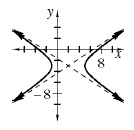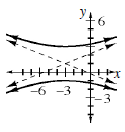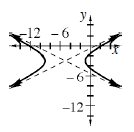### Home > A2C > Chapter 13 > Lesson 13.2.2 > Problem13-117

13-117.

Graph each of following relations. Some of the equations may need to be changed to a more useful form.

1. $\frac { ( x - 2 ) ^ { 2 } } { 9 } - \frac { ( y + 3 ) ^ { 2 } } { 4 } = 1$1. $\frac { y ^ { 2 } - 2 y + 1 } { 4 } - \frac { x ^ { 2 } + 6 x + 9 } { 25 } = 1$

Factor first.1. $4 ( x ^ { 2 } - 10 x + 25 ) - 16 ( y ^ { 2 } + 6 y + 9 ) = 64$

Factor, then divide.